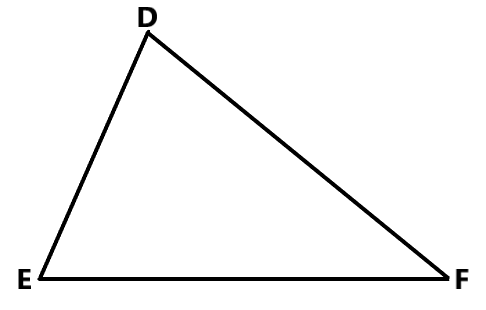Courses
Courses for Kids
Free study material
Free LIVE classes
More# Write answers to the following questions referring to the adjacent figure.i) Which is the angle opposite to the side DE?ii) Which is the side opposite to ${\angle{\, E \,}}$?iii) Which angle is included by side DE and DF?iv) Which side is included by ${\angle{\, E \,}}$ and ${\angle{\, F \,}}$ ?v) State the angles adjacent to side DE.

Last updated date: 26th Mar 2023
Total views: 207.6k
Views today: 1.84kVerified
207.6k+ views
Hint: Here in this question, we have to answer the given following sub questions using a given figure. To solve this, first observe the figure carefully and by referring to the figure we can answer the questions like opposite sides, opposite angles, angle included by side and adjacent side or angle easily.

Complete step by step solution:
In geometry, a triangle is a closed, two-dimensional shape with three straight sides and three angles.
Consider the given figure in the question:
In triangle $\Delta \,DEF$, line DE, EF and DF are the sides of the triangle and ${\angle{\, D \,}}$, ${\angle{\, E \,}}$ and ${\angle{\, F \,}}$ are the angles of $\Delta \,DEF$, then
Consider the questions

(i) Which is the angle opposite to the side DE?
In $\Delta \,DEF$,
DE is the side which opposite angle is ${\angle{\, {DFE} \,}}$ i.e., ${\angle{\, F \,}}$
Therefore, ${\angle{\, F \,}}$ is the angle opposite to the side DE.

(ii) Which is the side opposite to ${\angle{\, E \,}}$?
In $\Delta \,DEF$, DF is the side which opposite to the angle ${\angle{\, E \,}}$.

(iii) Which angle is included by side DE and DF?
In $\Delta \,DEF$, ${\angle{\, {EDF} \,}}$ i.e., ${\angle{\, D \,}}$ is the angle which includes or connects the sides DE and DF.

(iv) Which side is included by ${\angle{\, E \,}}$ and ${\angle{\, F \,}}$ ?
In $\Delta \,DEF$, Side EF is included by the angles ${\angle{\, E \,}}$ and ${\angle{\, F \,}}$.

(v) The angles adjacent to side DE,
In $\Delta \,DEF$, ${\angle{\, {DEF} \,}}$ and ${\angle{\, {EDF} \,}}$ i.e., ${\angle{\, E \,}}$ and ${\angle{\, D \,}}$ are the adjacent angles of the side DE.

Note:
While solving these type of questions, observe the carefully the sides and angles of the figure and remember some points are: adjacent side is the side next to the indicated angle or the side, adjacent angle is the two angles share a Common arm and a common vertex between them. And as usual the opposite side is the one across from a given angle or side.